# Electronics and Communication Engineering - Power Electronics

Exercise : Power Electronics - Section 6
31.
A thyristor circuit is feeding an R-L load. The turn on time can be reduced by
decreasing R
decreasing L
increasing L
decreasing R and L together
Explanation:
No answer description is available. Let's discuss.

32.
A single phase semiconverter is supplying RLE load and has a freewheeling diode across the load. The firing angle is a. The freewheeling diode conducts for
a° in each half cycle
(p - a)° in each cycle
a° in one full cycle
(p + a)° in each half cycle
Explanation:
No answer description is available. Let's discuss.

33.
In a full bridge inverter fed by a battery of voltage V, the rms value of fundamental component of output voltage is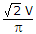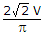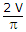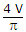Explanation:
No answer description is available. Let's discuss.

34.
In a single phase full wave regulator feeding a resistive load, the average current over one full cycle
is always zero
cannot be zero
may be sometimes zero
is always positive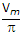(1 + cos a2)(2 + cos a2)(3 + cos a2)(4 + cos a2)